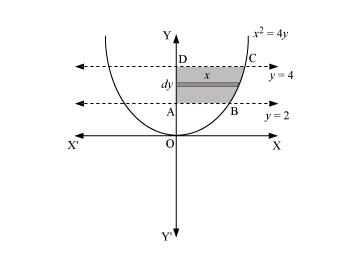Deepak Scored 45->99%ile with Bounce Back Crack Course. You can do it too!

# Find the area of the region bounded by

Question:

Find the area of the region bounded by $x^{2}=4 y, y=2, y=4$ and the $y$-axis in the first quadrant.

Solution:The area of the region bounded by the curve, $x^{2}=4 y, y=2$, and $y=4$, and the $y$-axis is the area $\mathrm{ABCD}$.

Area of $\mathrm{ABCD}=\int_{2}^{4} x d y$

$=\int_{2}^{4} 2 \sqrt{y} d y$

$=2 \int_{2}^{4} \sqrt{y} d y$

$=2\left[\frac{y^{\frac{3}{2}}}{\frac{3}{2}}\right]_{2}^{4}$

$=\frac{4}{3}\left[(4)^{\frac{3}{2}}-(2)^{\frac{3}{2}}\right]$

$=\frac{4}{3}[8-2 \sqrt{2}]$

$=\left(\frac{32-8 \sqrt{2}}{3}\right)$ units